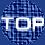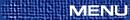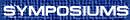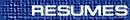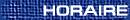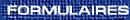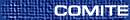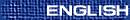Differential Geometry / Géométrie différentielle(J. Chen, Organizer) C. AREZZO, Università di Parma To be announced J. BRYAN, University of British Columbia, Vancouver Gromov-Witten theory of local Calabi-Yau's and TQFT We study the local Gromov-Witten theory of a curve in a Calabi-Yau 3-fold. We show this theory can be exhibited as a 1+1 dimensional topological quantum field theory. This theory is semi-simple and leads to nice closed formulas for the local Gromov-Witten invariants. MIHAIL COCOS, University of British Columbia On the heat-equation method of M. Gaffney The study of L2 harmonic forms on a complete Riemanian manifold is a very interesting and important subject. It has also found numerous applications in the field of Mathematical Physics and other related areas of Mathematics. As early as 1956 M. Gaffney in his PhD thesis at the University of Chicago proved that the Heat Equation for forms of arbitrary degree has solution for all time t > 0 and the solution is smooth (similar results were also obtained by F. Browder). He also showed that the ``heat flow'' generated by solving this equation preserves the De Rham cohomology class of the initial data, thus giving a topologycal method of constructing nontrivial L2 harmonic forms on certain manifolds with ``rich'' topology. Using Gaffney`s ``heat flow'' the author of the present paper gives a nice geometrical interpretation of the vanishing of the L2 cohomology space and also proves that a conformal change of metric in the case of the hyperbolic space has no effect on the L2 cohomology. A. FRASER, Brown University Fundamental groups of manifolds with positive isotropic curvature Positive isotropic curvature (PIC) is a natural and much studied curvature condition which includes manifolds with pointwise quarter-pinched sectional curvatures and manifolds with positive curvature operator. By results of Micallef and Moore there is only one topological type of compact simply connected manifold with PIC; namely any such manifold must be homeomorphic to a sphere. On the other hand, there is a large class of non-simply connected manifolds with PIC. An important open problem has been to understand the fundamental group of manifolds with PIC. In this talk we describe a new result in this direction. The techniques used involve minimal surfaces. JACQUES HURTUBISE, CRM G-bundles over an elliptic curve and dynamical r-matrices The r-matrix formalism of integrable systems ties in very nicely with the geometry of rigid bundles over a curve. Recently, through work of Felder, Etingopf and Varchenko, this formalism was extended to a particular non-rigid case, that of G-bundles over an elliptic curve. In joint work with Markman, the geometry of these systems is examined, and a description is given of the Poisson geometry, as well as a proof of integrability. X. LIU, Notre Dame Solving universal equations in Gromov-Witten invariants Universal equations for Gromov-Witten invariants are obtained from relations in homology classes of moduli spaces of stable curves. When the underlying manifolds have semisimple quantum cohomology, the generating functions of genus-2 Gromov-Witten invariants can be explicitly expressed in terms of genus-0 and genus-1 invariants by solving already existing universal equations. These equations can also be used to study the Virasoro conjecture. P. LU, Deparment of Mathematics, McMaster University, Hamilton, Ontario  L8S 4K1 Cheeger-Gromov type compactness theorem on oribifolds We will discuss the generalization of Cheeger-Gromov type compactness theorem to orbifolds. We will also discuss a compactness theorem on orbifolds in Ricci flow. D. MATESSI, Centre de Recherche Mathematiques, Universite de Montreal, Montreal, Quebec Families of special Lagrangian tori We will show some ways to construct isometric embeddings of one or two parameter families of special Lagrangian submanifolds. As an application we show that in dimension 3, the moduli space of special Lagrangian tori is not in general ``special Lagrangian'' in the sense of Hitchin, as it is instead in dimension 2. M. WANG, McMaster University, Hamilton, Ontario  L8S 4K1 Painleve analysis, Diophantine conditions, and special holonomy I will report on joint work with A. Dancer of Jesus College, Oxford. We apply the method of S. Kowalevskaya as further developed by Painlevé, and modern authors such as Ablowitz, Ramani, and Segur to a quadratic system associated to the cohomogeneity 1 Ricci-flat equations. The existence of ``large'' families of convergent Painlevé expansions in the cases under study leads to restrictions on the principal orbit type, including diophantine conditions on its dimension (e.g. existence of integral points on elliptic curves). Some of the diophantine conditions are related to metrics with special holonomy. R. WENTWORTH, Johns Hopkins University The Yang-Mills flow revisited This talk will outline work in progress with G. Daskalopoulos on the convergence properties of the Yang-Mills flow on holomorphic vector bundles over higher dimensional Kaehler manifolds. BAOZHONG YANG, Stanford University, Stanford, California  94305, USA Analytic aspects of gauge theories in higher dimensions I'll talk about some recent results on the analytical aspects for Yang-Mills theories in dimensions greater than 4. These include singular-compactness theorems, removable singularity theorems, as well structure of singularities.